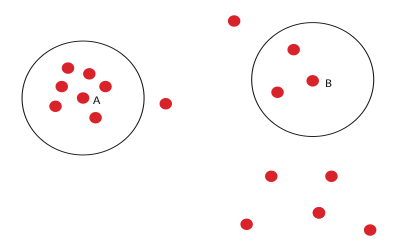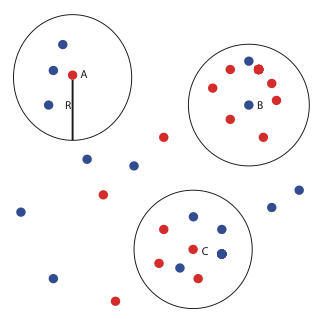# When Are Groups “Real”?

One of the key capabilities in topological data analysis is the identification of coherent subpopulations in complex data sets. Often these groups are based an outcome of interest.

For example, in a genomic data set based on a disease, where one has a sample from a number of subjects, the outcome might be death of the subject. In this case, one might create the network attached to the study, and then color each node by the fraction of subjects present in that node who do not survive. What then happens frequently is that there are a number of groups that can be visually identified as bright red, which one would then interpret as distinct forms of the disease, or distinct ways of dying from the disease.

Unfortunately, it is possible for such visually plausible groups to be created at random. It is important to formulate some criteria for the groups to be “real”, i.e. for which it is extremely unlikely that the groups could have been created at random.

One can formulate a strategy for developing such criteria based on an analogy. In epidemiology, one is often confronted with apparent disease clusters within a small geographic region.

It is well understood that within a large geographic unit, such localized clusters will often appear at random. It is important to understand whether or not a cluster is so concentrated that it is extremely unlikely that it could have appeared by chance. One approach to this question is to study the population density function on the geographic unit, and compare it with the density function based entirely on the disease cases. If one finds that the density function based on the disease cases is sufficiently elevated over the density function based on the whole population, one can often infer that the cluster is very unlikely to have occurred at random. One can formulate an analogous calculation for any group within a data set, to determine whether it is in a sense “sufficiently concentrated” to permit one to conclude that it would be extremely unlikely that it occurred at random.

In order to understand this idea, we first need to talk about what we mean by a data set.

A data set will be a set equipped with a dissimilarity measure, by which we mean an assignment of a number to each pair of data points which quantifies a notion of similarity, i.e. which is large when the two points are quite dissimilar to each other, and which is small when the points are similar. The mathematically precise term is a finite metric space, i.e. a set equipped with a distance function d that obeys the same formal properties as the familiar distance functions in the plane or in space.

This is a very useful abstraction, since it allows us to reason (in a wide variety of situations) by analogy with our intuition concerning ordinary geometry. It is also the case that nearly all data sets come equipped with natural distance functions of this type.

For example, numerical spreadsheet tables (with arbitrarily many columns) have several natural choices, based on a many-variable Pythagorean theorem or many-variable notions of angle. In data that is more “unstructured”, it is often necessary to construct such notions of distance to reflect an intuitive notion of similarity, but there are many tools and tricks to perform such constructions. In short, finite metric spaces cover most kinds of data that one deals with.

It is possible to develop notions of (or “proxies for”) density within the data set X. One primitive notion might be to fix a number R, and to assign to each element x in the data set the number of points in X whose distance from x is less than R. If there are many such points, then this is an indication that the point should be counted as dense, and if there are few, then it should be less dense.The two black circles include exactly the points whose distance from the center point is less than R.

The point labeled A has a count of 7 points within a distance of R, while the point labeled B has only three such points. A is “more dense” than B.

To construct the actual proxy, we will divide this count by the sum of the counts for all the points x in the metric space. This means we now have a probability mass function on the set X.

Note that although we are illustrating this notion for points in the plane, the idea applies to any metric space. There are numerous other proxies one could construct.

The proxy we have just described requires a fixed choice of the radius parameter R. If we choose R incorrectly, say so large that every pair of points has distance less than R, or so small that no pair of distinct points has distance less than R, then this is not a useful measure.

One way to get around this is to create a weighted sum that involves all the distances, for example that for each x, we add together the reciprocals of the distances to all points other than x. This sum will be large if there are a large number of points with small distance to x. We would then apply the averaging construction from above to these values to get a probability mass function on X. There is no choice of parameter R involved in this approach.

Let us suppose that we have now chosen the first proxy above, in which we count the number of points with distance less than or equal to R from x, for a fixed value of R, and perform the averaging construction.

Suppose in addition that we are given a subgroup S within X, perhaps characterized by an outcome of interest. We might be considering a data set of patients with a number of clinical and non-clinical variables, with a group characterized by the outcome of survival. Or, we might be considering a data set gathered from measurements on a collection of devices, with the outcome being failure. We want to understand the degree to which something systematic or non-random is happening to enhance survival or to cause failure of a device.

One way to study this is to construct another density proxy, in this case based only on the points in S. It is obtained by counting the number of points in S whose distance from x is less than R, and then dividing by the sum of these values for all points in X. This quantity is in a sense reflective of the density of points of S in X. We can now compute the relative density proxy to be the quotient of the proxy for S-density by the proxy for X density. High values of this statistic indicate elevated density for S relative to the background density given from X.In the example above, blue dots indicate members of the group and red dots indicate non-members. Each of the circles have radius R.   There are 15 group members and 15 non-group members.

The circle centered at A is clearly the least dense overall, but the density of points in the group is larger than one would expect.

On the other hand, the circle centered at B is relatively dense in the overall measure of density, but is very low in the density of group-members.

The circle centered at C is of reasonably high density overall, and its group-member density is at a correspondingly high level.

The conclusion here might be that non-random phenomena are accounting for unusually high (respectively unusually low) presence of group members in the circles centered at A and B, respectively, while it appears that group C could be accounted for as random distribution of the members of the group. One can make this quantitative by recording for each of the circles the fraction of the points that are in the subgroup. So, for the circle centered at A, we would record the fraction 3/4, for the circle centered at B, the result would be 2/9, and for the circle centered at C we would have 1/2.

In order to construct numerical criteria for evaluating the “non-randomness” of a group, one can devise Monte Carlo sampling schemes for subsets of size 15 within the 30 total points in the set. To do this, one devises a probability mass function of the subsets consisting of 15 members based on the density proxy for X. By sampling from this distribution, and evaluating the corresponding fractions for each of the circles in question, we can for example begin to assess how frequently a fraction greater than 3/4 occurs for the circle centered at A and how frequently a fraction less than 2/9 occurs for the circle centered at B.

This permits us to make judgments about how likely it is that a given group could have occurred at random, and more generally which parts of the group are less likely to have been achieved at random.

In summary, we have formulated a way to make precise statements that give answers to the intuitive query “is this group real?”. The formulation is in terms of concentration of elements of a given outcome within the background concentration in the data set.

These notions should be very useful in various inference questions arising in the analysis of data.